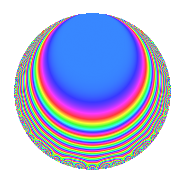# Properties

 Label 800.1.bhLevel 800 Weight 1 Character orbit bh Rep. character $$\chi_{800}(31,\cdot)$$ Character field $$\Q(\zeta_{10})$$ Dimension 8 Newforms 1 Sturm bound 120 Trace bound 0

# Related objects

## Defining parameters

 Level: $$N$$ = $$800 = 2^{5} \cdot 5^{2}$$ Weight: $$k$$ = $$1$$ Character orbit: $$[\chi]$$ = 800.bh (of order $$10$$ and degree $$4$$) Character conductor: $$\operatorname{cond}(\chi)$$ = $$100$$ Character field: $$\Q(\zeta_{10})$$ Newforms: $$1$$ Sturm bound: $$120$$ Trace bound: $$0$$

## Dimensions

The following table gives the dimensions of various subspaces of $$M_{1}(800, [\chi])$$.

Total New Old
Modular forms 56 8 48
Cusp forms 24 8 16
Eisenstein series 32 0 32

The following table gives the dimensions of subspaces with specified projective image type.

$$D_n$$ $$A_4$$ $$S_4$$ $$A_5$$
Dimension 0 0 0 8

## Trace form

 $$8q - 2q^{5} + O(q^{10})$$ $$8q - 2q^{5} + 6q^{13} + 4q^{21} - 2q^{25} - 6q^{29} + 2q^{37} - 4q^{49} + 2q^{53} - 4q^{57} - 2q^{61} - 4q^{65} + 2q^{69} - 2q^{73} + 2q^{81} - 8q^{93} + 4q^{97} + O(q^{100})$$

## Decomposition of $$S_{1}^{\mathrm{new}}(800, [\chi])$$ into irreducible Hecke orbits

Label Dim. $$A$$ Field Image CM RM Traces $q$-expansion
$$a_2$$ $$a_3$$ $$a_5$$ $$a_7$$
800.1.bh.a $$8$$ $$0.399$$ $$\Q(\zeta_{20})$$ $$A_{5}$$ None None $$0$$ $$0$$ $$-2$$ $$0$$ $$q-\zeta_{20}^{7}q^{3}+\zeta_{20}^{4}q^{5}+(\zeta_{20}+\zeta_{20}^{9}+\cdots)q^{7}+\cdots$$

## Decomposition of $$S_{1}^{\mathrm{old}}(800, [\chi])$$ into lower level spaces

$$S_{1}^{\mathrm{old}}(800, [\chi]) \cong$$ $$S_{1}^{\mathrm{new}}(100, [\chi])$$$$^{\oplus 4}$$